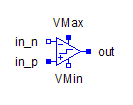Multiphase Ideal Closing Switch - MapleSim Help

Multiphase Ideal Closing Switch

Multiphase ideal closerDescription The Multiphase Ideal Closing Switch (or Ideal Closing Switch) component contain $m$ single-phase ideal closing switch models, each connected between corresponding phases of ${\mathrm{plug}}_{p}$ and ${\mathrm{plug}}_{n}$, with the control from the corresponding signal in the $\mathrm{control}$ vector input. A vector of optional thermal ports, one for each switch, is enabled if Use Heat Port is true.Connections

 Name Description Modelica ID ${\mathrm{plug}}_{p}$ Positive $m$-phase plug plug_p ${\mathrm{plug}}_{n}$ Negative $m$-phase plug plug_n $\mathrm{control}$ Boolean vector input control $\mathrm{Heat Port}$ Optional thermal ports heatPortParameters

 Name Default Units Description Modelica ID $m$ $3$ Number of phases m ${R}_{\mathrm{on}}$ * $1·{10}^{-5}$ $\mathrm{\Omega }$ Closed switch resistance Ron ${G}_{\mathrm{off}}$ * FLOATE(1,-5) $S$ Opened switch conductance Goff Use Heat Port $\mathrm{false}$ True (checked) means heat port is enabled useHeatPort

* Starred values are vector parameters; the default is $\mathrm{fill}\left(x,m\right)$, which creates a vector with $m$ entries of $x$.Modelica Standard Library The component described in this topic is from the Modelica Standard Library. To view the original documentation, which includes author and copyright information, click here.